# C++ Integer Data Type

Data types describe the kind of data so that computer can store or manipulate them appropriately. This is the reason to declare a data type in C++ programming.

Data types are also necessary because data items such as numbers are used in expressions. If there is a mismatch, for example – integer and real numbers – in an expression then the computer must be able to resolve it. C++ allows the following data types.

• Integer
• Float
• Double
• Char

## Memory Organization

All data is stored in memory which is organized in bytes. A single byte is of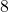bits.

The integer data type takes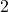or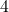bytes to store information. This means it takes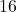or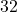bit space.

## Integer Data Types

The integers are negative and positive numbers. C++ add modifiers like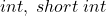to integers to describe size and range of values of the integer types. The list of modifiers is given below.

1. int
2. long int
3. short int
4. signed int
5. unsigned int

For example

short int = 2 bytes = 16 bits

The integers have both positive and negative values and each bit position takes two values –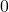or.

Therefore, its range is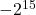to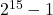The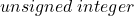type takes only positive values and the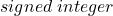value takes both positive and negative values. All the integer types takebytes of space except the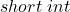which takes onlybytes of information.

## An Example Program

// Program to display the size of integer data types
#include <iostream.h>
int main()
{
int a = 10;
short int b = 12;
long int c = 100;
unsigned int d = 30;
signed int e = 32;

cout << sizeof(a) << "Bytes" << endl;
cout << sizeof(b) << "Bytes" << endl;
cout << sizeof(c) << "Bytes" << endl;
cout << sizeof(d) << "Bytes" << endl;
cout << sizeof(e) << "Bytes" << endl;
system("pause");
return 0;
}

## Output

4 Bytes
2 Bytes
4 Bytes
4 Bytes
4 Bytes

The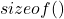function takes the variable as an argument and then output the byte size of the variable. The integer variable has a size ofbytes on my windows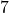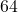bit machine. The size of the integer variable may be different on a different machine.